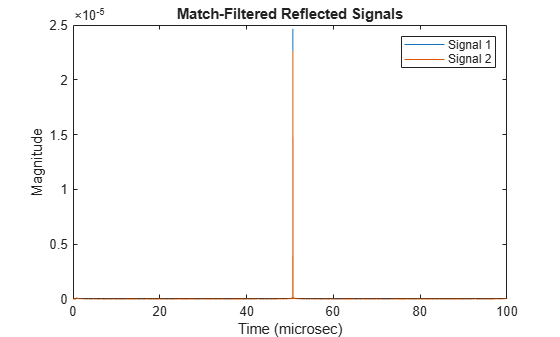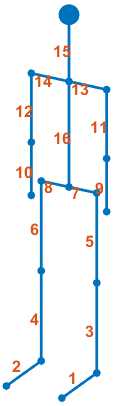# move

Position and velocity of walking pedestrian

## Syntax

``[BPPOS,BPVEL,BPAX] = move(pedestrian,T,ANGH)``

## Description

example

````[BPPOS,BPVEL,BPAX] = move(pedestrian,T,ANGH)` returns the position, `BPPOS`, velocity, `BPVEL`, and orientation axes, `BPAX`, of body segments of a moving pedestrian. The object then simulates the walking motion for the next duration, specified in `T`. `ANGH` specifies the current heading angle.```

## Examples

collapse all

Compute the reflected radar signal from a pedestrian moving along the x-axis away from the origin. The radar operates at 24 GHz and is located at the origin. The pedestrian is initially 100 meters from the radar. Transmit a linear FM waveform having a 300 MHz bandwidth. The reflected signal is captured at the moment the pedestrian starts to move and at two seconds into the motion.

Create a linear FM waveform and a free space channel to propagate the waveform.

```c = physconst('Lightspeed'); bw = 300.0e6; fs = bw; fc = 24.0e9; wav = phased.LinearFMWaveform('SampleRate',fs,'SweepBandwidth',bw); x = wav(); channel = phased.FreeSpace('OperatingFrequency',fc,'SampleRate',fs, ... 'TwoWayPropagation',true);```

Create the pedestrian object. Set the initial position of the pedestrian to 100 m on the x-axis with initial heading along the positive x-direction. The pedestrian height is 1.8 m and the pedestrian is walking at 0.5 meters per second.

```pedest = backscatterPedestrian( 'Height',1.8, ... 'OperatingFrequency',fc,'InitialPosition',[100;0;0], ... 'InitialHeading',0,'WalkingSpeed',0.5);```

The first call to the `move` function returns the initial position, initial velocity, and initial orientation of all body segments and then advances the pedestrian motion two seconds ahead.

`[bppos,bpvel,bpax] = move(pedest,2,0);`

Transmit the first pulse to the pedestrian. Create 16 replicas of the signal and propagate them to the positions of the pedestrian body segments. Use the `rangeangle` function to compute the arrival angle of each replica at the corresponding body segment. Then use the `reflect` function to return the coherent sum of all the reflected signals from the body segments at the pedestrian initial position.

```radarpos = [0;0;0]; xp = channel(repmat(x,1,16),radarpos,bppos,[0;0;0],bpvel); [~,ang] = rangeangle(radarpos,bppos,bpax); y0 = reflect(pedest,xp,ang);```

Obtain the position, velocity, and orientation of each body segment then advance the pedestrian motion another two seconds.

`[bppos,bpvel,bpax] = move(pedest,2,0);`

Transmit and propagate the second pulse to the new position of the pedestrian.

```radarpos = [0;0;0]; xp = channel(repmat(x,1,16),radarpos,bppos,[0;0;0],bpvel); [~,ang] = rangeangle(radarpos,bppos,bpax); y1 = reflect(pedest,xp,ang);```

Match-filter and plot both of the reflected pulses. The plot shows the increased delay of the matched filter output as the pedestrian walks away.

```filter = phased.MatchedFilter('Coefficients',getMatchedFilter(wav)); ymf = filter([y0 y1]); t = (0:size(ymf,1)-1)/fs; plot(t*1e6,abs(ymf)) xlabel('Time (microsec)') ylabel('Magnitude') title('Match-Filtered Reflected Signals') legend('Signal 1','Signal 2')```Zoom in and show the time delays for each signal.

```plot(t*1e6,abs(ymf)) xlabel('Time (microsec)') ylabel('Magnitude') title('Matched-Filtered Reflected Signals') axis([50.65 50.7 0 .0026]) legend('Signal 1','Signal 2')```## Input Arguments

collapse all

Pedestrian target model, specified as a `backscatterPedestrian` object.

Duration of next walking interval, specified as a positive scalar. Units are in seconds.

Example: `0.75`

Data Types: `double`

Heading of the pedestrian, specified as a scalar. Heading is measured in the xy-plane from the x-axis towards the y-axis. Units are in degrees.

Example: `-34`

Data Types: `double`

## Output Arguments

collapse all

Positions of body segments, returned as a real-valued 3-by-16 matrix. Each column represents the Cartesian position, `[x;y;z]`, of one of 16 body segments. Units are in meters. See Body Segment Indices for the column representing the position of each body segment.

Data Types: `double`

Velocity of body segments, returned as a real-valued 3-by-16 matrix. Each column represents the Cartesian velocity vector, `[vx;vy;vz]`, of one of 16 body segments. Units are in meters per second. See Body Segment Indices for the column representing the velocity of each body segment.

Data Types: `double`

Orientation axes of body segments, returned as a real-valued 3-by-3-by-16 array. Each page represents the 3-by-3 orientation axes of one of 16 body segments. Units are dimensionless. See Body Segment Indices for the page representing the orientation of each body segment.

Data Types: `double`

collapse all

### Body Segment Indices

Body segment indices define which columns in `BPPOS` and `BPVEL` contain the position and velocity data for a specific body segment. The indices also point to the page of `BPAX` containing the orientation matrix for a specific body segment. For example, column three of `BPPOS` contains the 3-D position of the left lower leg. Page three of `BPAX` contains the orientation matrix of the left lower leg.

Body SegmentIndex
Left foot1Right foot2
Left lower leg3
Right lower leg4
Left upper leg5
Right upper leg6
Left hip7
Right hip8
Left lower arm9
Right lower arm10
Left upper arm11
Right upper arm12
Left shoulder13
Right shoulder14